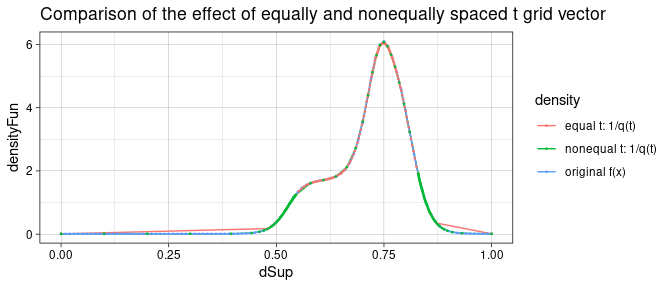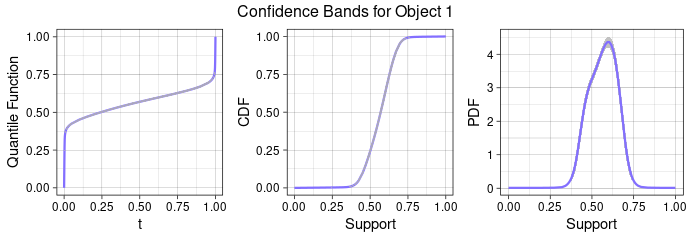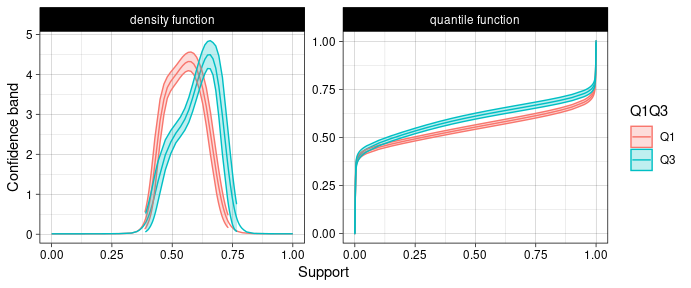# Introduction to the WRI Package

The Wasserstein Regression Inference (WRI) package performs statistical inference in density regression, in which the response is a one-dimensional probability density and predictors are scalars. The package implements methods proposed in the paper, Wasserstein F-tests and confidence bands for the Frechet regression of density response curves. Link to Paper on Arxiv.

Install and library WRI using:

# install.packages('WRI')
library(WRI)

We will use dataset strokeCTdensity to illustrate functions in WRI package. This dataset contains clinical, radiological scalar variables, and hematoma density curves for 393 stroke patients.

data(strokeCTdensity)
?strokeCTdensity

predictor = strokeCTdensity$predictors dSup = strokeCTdensity$densitySupport
densityCurves = strokeCTdensity$densityCurve # 1 Wasserstein Regression wass_regress is the estimation function which works similar to lm. To compute the fitted values, it requires a formula, response and predictor data. We give explanation of other arguments below. • Ytype: whether the response matrix Ymat contains 'quantile' or 'density' functions. • Sup: the common grid for density/quantile functions in Ymat. ### 1.0.1 The effect of Sup grid vector when Ytype == 'quantile' Since most derivation in WRI works in the space of quantile functions and its derivatives, the probability density functions are converted into quantile functions. However, the transformation will result in certain deviation between the original density function and $$1/q(t)$$, where $$q(t) = Q'(t), t = F(x)$$. Note that it is $$q(t)$$’s that are directly used in the WRI functions. Below we set t as equally spaced grid vector and nonequally spaced vector, which is denser near the boundary to compare the resulting $$1/q(t)$$. equal_t = den2Q_qd(densityCurves, dSup, t_vec = seq(0, 1, length.out = 120)) nonequal_t = den2Q_qd(densityCurves, dSup, t_vec = unique(c(seq(0, 0.05, 0.001), seq(0.05, 0.95, 0.05), seq(0.95, 1, 0.001))))When the quantile support vector is finer near the boundary, $$1/q(t)$$ is closer to original density function $$f(x)$$. Thus, when user inputs density functions as response curves, i.e. Ytype == 'density', the support for quantile functions is set as nonequal_t. ### 1.0.2 Usage of wass_regress function The density curves and predictor variables are input into wass_regress separately, as illustrated below. res = wass_regress(rightside_formula = ~., Xfit_df = predictor, Ytype = 'density', Ymat = densityCurves, Sup = dSup) The wass_regress function returns a WRI object. This object can be used with the other functions in this package to run hypothesis tests, calculate Wasserstein $$R^2$$, and compute confidence bands. ## 1.1 Summary The summary method for WRI objects combines the global F-test, Wassertstein $$R^2$$, and partial F-tests for individual effects into one easily-readable output. summary(res) #> Call: #> wass_regress(rightside_formula = ~., Xfit_df = predictor, Ytype = "density", #> Ymat = densityCurves, Sup = dSup) #> #> Partial F test for individual effects: #> #> F-stat p-value(truncated) p-value(satterthwaite) #> log_b_vol 0.256 0.002 0.000 #> b_shapInd 0.062 0.002 0.000 #> midline_shift 0.035 0.002 0.000 #> weight 0.028 0.002 0.000 #> DM 0.012 0.028 0.022 #> AntiPt 0.007 0.114 0.116 #> age 0.000 0.914 0.885 #> B_TimeCT 0.000 0.920 0.857 #> Warfarin 0.003 0.365 0.372 #> #> Wasserstein R-squared: 0.224 #> F-statistic (by Satterthwaite method): 141.008 on 11.218 DF, p-value: 1.356e-24 ### 1.1.1 Wasserstein Coefficient of Determination, $$R^2$$ The Wasserstein coefficient of determination, $$R^2$$ can be calculated with wass_R2(res). The formula for the Wasserstein $$R^2$$ is as follows: $R^2=1-\frac{\sum^n_{i=1} d_W^2(f_{i}, \hat{f}_i)}{\sum^n_{i=1} d_W^2(f_{i}, \overline{f_{i}})},$ Where $$\overline{f_{i}}$$ is the unconditional Wasserstein mean estimate and $$\hat{f}_i$$ is the conditional mean estimate. This value represents the fraction of Wasserstein variability explained by the model, and can therefore be used to assess the goodness of fit for a model. ## 1.2 Hypothesis Testing ### 1.2.1 Global F-test globalFtest function performs the global F-tests. It provides four methods of computing the p-value, two (truncated and satterthwaite) through asymptotic analysis and two resampling techniques (permutation and bootstrap). Please note that the resampling methods can be slow. • Setting permutation = TRUE will also compute permutation p-value. The number of permutation samples can be controlled with the numPermu argument. • Setting bootstrap = TRUE will also compute bootstrap p-value. The number of bootstrap samples can be controlled with the numBoot argument. Note on Degrees of Freedom : The degrees of freedom are approximated by a chi-square distribution, so there is only 1 degree of freedom for our F-statistic. This is done because the F-statistic is asymptotically equivalent to a chi-squared distribution. globalF_res = globalFtest(res, alpha = 0.05, permutation = TRUE, numPermu = 200) kable(globalF_res$summary_df, digits = 3)
method statistic critical_value p_value
truncated 0.338 0.049 0.002
satterthwaite 0.338 0.048 0.000
permutation 0.338 0.062 0.005
sprintf('The wasserstein F-statistic is %.3f on %.3f degrees of freedom', globalF_res$wasserstein.F_stat, globalF_res$chisq_df)
#>  "The wasserstein F-statistic is 141.008 on 11.218 degrees of freedom"

### 1.2.2 Partial F-test

partialFtest can be used to test individual effects or submodel fits. Using the stroke data as an example, we test whether the clinical variables are significant for head CT hematoma densities when radiological variables are in the model.

# the reduced model only has four radiological variables
reduced_res = wass_regress(~ log_b_vol + b_shapInd + midline_shift + B_TimeCT, Xfit_df = predictor, Ymat = densityCurves, Ytype = 'density', Sup = dSup)
full_res = wass_regress(rightside_formula = ~., Xfit_df = predictor, Ymat = densityCurves, Ytype = 'density', Sup = dSup)

partialFtable = partialFtest(reduced_res, full_res, alpha = 0.05)
kable(partialFtable, digits = 3)
method statistic critical_value p_value
95% truncated 0.056 0.100 0.828
satterthwaite 0.056 0.099 0.839

With p-value greater than 0.05, we are confident to conclude that when radiological variables are in the model, clinical variables are not significant for explaining the variance in head CT hematoma densities.

## 1.3 Confidence Bands

### 1.3.1 Usage of confidenceBands function

The confidenceBands function computes the intrinsic Wasserstein$$-\infty$$ bands and Wasserstein density bands. In the function, these refer to quantile band and density band respectively, which are controlled by type argument (options are ‘quantile’, ‘density’ or ‘both’). By default, the function visualizes confidence bands for one object. But it allows to compute $$k$$ confidence bands simultaneously if a $$k \times p$$ dataframe Xpred_df is provided. All the results, including upper and lower bounds, predicted density function etc, are returned in a list.

xpred = colMeans(predictor)
confidence_Band = confidenceBands(res, Xpred_df = xpred, type = 'both')### 1.3.2 Investigate the effect of hematoma volume

We set log(hematoma volume) equal to the first quartile (Q1) or third quartile (Q3) of the observed values, with all other predictors set at their mean (for continuous variables) or mode (for binary variables). Then compare the CT hematoma densities in these two cases.

mean_Mode <- function(vec) {
return(ifelse(length(unique(vec)) < 3, modeest::mfv(vec), mean(vec)))
}
mean_mode_vec = apply(predictor, 2, mean_Mode)
predictorDF = rbind(mean_mode_vec, mean_mode_vec)
predictorDF[ , 1] = quantile(predictor$log_b_vol, probs = c(1/4, 3/4)) res_cb = confidenceBands(res, predictorDF, level = 0.95, delta = 0.01, type = 'both', figure = F) m = ncol(res_cb$quan_list$Q_lx) na.mat = matrix(NA, nrow = 2, ncol = m - ncol(res_cb$den_list$f_lx)) cb_plot_df = with(res_cb, data.frame( fun = rep(c('quantile function', 'density function'), each = 2*m), Q1Q3 = rep(rep(c('Q1', 'Q3'), each = m), 2), value_m = c(as.vector(t(quan_list$Qpred)), as.vector(t(cbind(cdf_list$fpred)))), value_u = c(as.vector(t(quan_list$Q_ux)), as.vector(t(cbind(den_list$f_ux, na.mat)))), value_l = c(as.vector(t(quan_list$Q_lx)), as.vector(t(cbind(den_list$f_lx, na.mat)))), support_full = c(rep(quan_list$t, 2), as.vector(t(cbind(cdf_list$Fsup)))), support_short = c(rep(quan_list$t, 2), as.vector(t(cbind(den_list\$Qpred, na.mat))))
))

ggplot(data = cb_plot_df, aes(color = Q1Q3)) +
theme_linedraw()+
geom_line(aes(x = support_full, y = value_m)) +
geom_ribbon(aes(x = support_short, ymin = value_l, ymax = value_u, fill = Q1Q3), alpha = 0.25) +
facet_wrap( ~ fun, scales = "free_y") +
ylab('Confidence band') +
xlab('Support')